/scikit-learn

# sklearn.tree.DecisionTreeClassifier

`class sklearn.tree.DecisionTreeClassifier(criterion=’gini’, splitter=’best’, max_depth=None, min_samples_split=2, min_samples_leaf=1, min_weight_fraction_leaf=0.0, max_features=None, random_state=None, max_leaf_nodes=None, min_impurity_decrease=0.0, min_impurity_split=None, class_weight=None, presort=False)` [source]

A decision tree classifier.

Read more in the User Guide.

Parameters: `criterion : string, optional (default=”gini”)` The function to measure the quality of a split. Supported criteria are “gini” for the Gini impurity and “entropy” for the information gain. `splitter : string, optional (default=”best”)` The strategy used to choose the split at each node. Supported strategies are “best” to choose the best split and “random” to choose the best random split. `max_depth : int or None, optional (default=None)` The maximum depth of the tree. If None, then nodes are expanded until all leaves are pure or until all leaves contain less than min_samples_split samples. `min_samples_split : int, float, optional (default=2)` The minimum number of samples required to split an internal node: If int, then consider `min_samples_split` as the minimum number. If float, then `min_samples_split` is a fraction and `ceil(min_samples_split * n_samples)` are the minimum number of samples for each split. Changed in version 0.18: Added float values for fractions. `min_samples_leaf : int, float, optional (default=1)` The minimum number of samples required to be at a leaf node. A split point at any depth will only be considered if it leaves at least `min_samples_leaf` training samples in each of the left and right branches. This may have the effect of smoothing the model, especially in regression. If int, then consider `min_samples_leaf` as the minimum number. If float, then `min_samples_leaf` is a fraction and `ceil(min_samples_leaf * n_samples)` are the minimum number of samples for each node. Changed in version 0.18: Added float values for fractions. `min_weight_fraction_leaf : float, optional (default=0.)` The minimum weighted fraction of the sum total of weights (of all the input samples) required to be at a leaf node. Samples have equal weight when sample_weight is not provided. `max_features : int, float, string or None, optional (default=None)` The number of features to consider when looking for the best split: If int, then consider `max_features` features at each split. If float, then `max_features` is a fraction and `int(max_features * n_features)` features are considered at each split. If “auto”, then `max_features=sqrt(n_features)`. If “sqrt”, then `max_features=sqrt(n_features)`. If “log2”, then `max_features=log2(n_features)`. If None, then `max_features=n_features`. Note: the search for a split does not stop until at least one valid partition of the node samples is found, even if it requires to effectively inspect more than `max_features` features. `random_state : int, RandomState instance or None, optional (default=None)` If int, random_state is the seed used by the random number generator; If RandomState instance, random_state is the random number generator; If None, the random number generator is the RandomState instance used by `np.random`. `max_leaf_nodes : int or None, optional (default=None)` Grow a tree with `max_leaf_nodes` in best-first fashion. Best nodes are defined as relative reduction in impurity. If None then unlimited number of leaf nodes. `min_impurity_decrease : float, optional (default=0.)` A node will be split if this split induces a decrease of the impurity greater than or equal to this value. The weighted impurity decrease equation is the following: ```N_t / N * (impurity - N_t_R / N_t * right_impurity - N_t_L / N_t * left_impurity) ``` where `N` is the total number of samples, `N_t` is the number of samples at the current node, `N_t_L` is the number of samples in the left child, and `N_t_R` is the number of samples in the right child. `N`, `N_t`, `N_t_R` and `N_t_L` all refer to the weighted sum, if `sample_weight` is passed. New in version 0.19. `min_impurity_split : float,` Threshold for early stopping in tree growth. A node will split if its impurity is above the threshold, otherwise it is a leaf. Deprecated since version 0.19: `min_impurity_split` has been deprecated in favor of `min_impurity_decrease` in 0.19 and will be removed in 0.21. Use `min_impurity_decrease` instead. `class_weight : dict, list of dicts, “balanced” or None, default=None` Weights associated with classes in the form `{class_label: weight}`. If not given, all classes are supposed to have weight one. For multi-output problems, a list of dicts can be provided in the same order as the columns of y. Note that for multioutput (including multilabel) weights should be defined for each class of every column in its own dict. For example, for four-class multilabel classification weights should be [{0: 1, 1: 1}, {0: 1, 1: 5}, {0: 1, 1: 1}, {0: 1, 1: 1}] instead of [{1:1}, {2:5}, {3:1}, {4:1}]. The “balanced” mode uses the values of y to automatically adjust weights inversely proportional to class frequencies in the input data as `n_samples / (n_classes * np.bincount(y))` For multi-output, the weights of each column of y will be multiplied. Note that these weights will be multiplied with sample_weight (passed through the fit method) if sample_weight is specified. `presort : bool, optional (default=False)` Whether to presort the data to speed up the finding of best splits in fitting. For the default settings of a decision tree on large datasets, setting this to true may slow down the training process. When using either a smaller dataset or a restricted depth, this may speed up the training. `classes_ : array of shape = [n_classes] or a list of such arrays` The classes labels (single output problem), or a list of arrays of class labels (multi-output problem). `feature_importances_ : array of shape = [n_features]` Return the feature importances. `max_features_ : int,` The inferred value of max_features. `n_classes_ : int or list` The number of classes (for single output problems), or a list containing the number of classes for each output (for multi-output problems). `n_features_ : int` The number of features when `fit` is performed. `n_outputs_ : int` The number of outputs when `fit` is performed. `tree_ : Tree object` The underlying Tree object. Please refer to `help(sklearn.tree._tree.Tree)` for attributes of Tree object and Understanding the decision tree structure for basic usage of these attributes.

#### Notes

The default values for the parameters controlling the size of the trees (e.g. `max_depth`, `min_samples_leaf`, etc.) lead to fully grown and unpruned trees which can potentially be very large on some data sets. To reduce memory consumption, the complexity and size of the trees should be controlled by setting those parameter values.

The features are always randomly permuted at each split. Therefore, the best found split may vary, even with the same training data and `max_features=n_features`, if the improvement of the criterion is identical for several splits enumerated during the search of the best split. To obtain a deterministic behaviour during fitting, `random_state` has to be fixed.

#### References

  L. Breiman, J. Friedman, R. Olshen, and C. Stone, “Classification and Regression Trees”, Wadsworth, Belmont, CA, 1984.
  T. Hastie, R. Tibshirani and J. Friedman. “Elements of Statistical Learning”, Springer, 2009.
  L. Breiman, and A. Cutler, “Random Forests”, http://www.stat.berkeley.edu/~breiman/RandomForests/cc_home.htm

#### Examples

```>>> from sklearn.datasets import load_iris
>>> from sklearn.model_selection import cross_val_score
>>> from sklearn.tree import DecisionTreeClassifier
>>> clf = DecisionTreeClassifier(random_state=0)
>>> iris = load_iris()
>>> cross_val_score(clf, iris.data, iris.target, cv=10)
...
...
array([ 1.     ,  0.93...,  0.86...,  0.93...,  0.93...,
0.93...,  0.93...,  1.     ,  0.93...,  1.      ])
```

#### Methods

 `apply`(X[, check_input]) Returns the index of the leaf that each sample is predicted as. `decision_path`(X[, check_input]) Return the decision path in the tree `fit`(X, y[, sample_weight, check_input, …]) Build a decision tree classifier from the training set (X, y). `get_params`([deep]) Get parameters for this estimator. `predict`(X[, check_input]) Predict class or regression value for X. `predict_log_proba`(X) Predict class log-probabilities of the input samples X. `predict_proba`(X[, check_input]) Predict class probabilities of the input samples X. `score`(X, y[, sample_weight]) Returns the mean accuracy on the given test data and labels. `set_params`(**params) Set the parameters of this estimator.
`__init__(criterion=’gini’, splitter=’best’, max_depth=None, min_samples_split=2, min_samples_leaf=1, min_weight_fraction_leaf=0.0, max_features=None, random_state=None, max_leaf_nodes=None, min_impurity_decrease=0.0, min_impurity_split=None, class_weight=None, presort=False)` [source]
`apply(X, check_input=True)` [source]

Returns the index of the leaf that each sample is predicted as.

New in version 0.17.

Parameters: `X : array_like or sparse matrix, shape = [n_samples, n_features]` The input samples. Internally, it will be converted to `dtype=np.float32` and if a sparse matrix is provided to a sparse `csr_matrix`. `check_input : boolean, (default=True)` Allow to bypass several input checking. Don’t use this parameter unless you know what you do. `X_leaves : array_like, shape = [n_samples,]` For each datapoint x in X, return the index of the leaf x ends up in. Leaves are numbered within `[0; self.tree_.node_count)`, possibly with gaps in the numbering.
`decision_path(X, check_input=True)` [source]

Return the decision path in the tree

New in version 0.18.

Parameters: `X : array_like or sparse matrix, shape = [n_samples, n_features]` The input samples. Internally, it will be converted to `dtype=np.float32` and if a sparse matrix is provided to a sparse `csr_matrix`. `check_input : boolean, (default=True)` Allow to bypass several input checking. Don’t use this parameter unless you know what you do. `indicator : sparse csr array, shape = [n_samples, n_nodes]` Return a node indicator matrix where non zero elements indicates that the samples goes through the nodes.
`feature_importances_`

Return the feature importances.

The importance of a feature is computed as the (normalized) total reduction of the criterion brought by that feature. It is also known as the Gini importance.

Returns: `feature_importances_ : array, shape = [n_features]`
`fit(X, y, sample_weight=None, check_input=True, X_idx_sorted=None)` [source]

Build a decision tree classifier from the training set (X, y).

Parameters: `X : array-like or sparse matrix, shape = [n_samples, n_features]` The training input samples. Internally, it will be converted to `dtype=np.float32` and if a sparse matrix is provided to a sparse `csc_matrix`. `y : array-like, shape = [n_samples] or [n_samples, n_outputs]` The target values (class labels) as integers or strings. `sample_weight : array-like, shape = [n_samples] or None` Sample weights. If None, then samples are equally weighted. Splits that would create child nodes with net zero or negative weight are ignored while searching for a split in each node. Splits are also ignored if they would result in any single class carrying a negative weight in either child node. `check_input : boolean, (default=True)` Allow to bypass several input checking. Don’t use this parameter unless you know what you do. `X_idx_sorted : array-like, shape = [n_samples, n_features], optional` The indexes of the sorted training input samples. If many tree are grown on the same dataset, this allows the ordering to be cached between trees. If None, the data will be sorted here. Don’t use this parameter unless you know what to do. `self : object`
`get_params(deep=True)` [source]

Get parameters for this estimator.

Parameters: `deep : boolean, optional` If True, will return the parameters for this estimator and contained subobjects that are estimators. `params : mapping of string to any` Parameter names mapped to their values.
`predict(X, check_input=True)` [source]

Predict class or regression value for X.

For a classification model, the predicted class for each sample in X is returned. For a regression model, the predicted value based on X is returned.

Parameters: `X : array-like or sparse matrix of shape = [n_samples, n_features]` The input samples. Internally, it will be converted to `dtype=np.float32` and if a sparse matrix is provided to a sparse `csr_matrix`. `check_input : boolean, (default=True)` Allow to bypass several input checking. Don’t use this parameter unless you know what you do. `y : array of shape = [n_samples] or [n_samples, n_outputs]` The predicted classes, or the predict values.
`predict_log_proba(X)` [source]

Predict class log-probabilities of the input samples X.

Parameters: `X : array-like or sparse matrix of shape = [n_samples, n_features]` The input samples. Internally, it will be converted to `dtype=np.float32` and if a sparse matrix is provided to a sparse `csr_matrix`. `p : array of shape = [n_samples, n_classes], or a list of n_outputs` such arrays if n_outputs > 1. The class log-probabilities of the input samples. The order of the classes corresponds to that in the attribute `classes_`.
`predict_proba(X, check_input=True)` [source]

Predict class probabilities of the input samples X.

The predicted class probability is the fraction of samples of the same class in a leaf.

`check_input : boolean, (default=True)`
Allow to bypass several input checking. Don’t use this parameter unless you know what you do.
Parameters: `X : array-like or sparse matrix of shape = [n_samples, n_features]` The input samples. Internally, it will be converted to `dtype=np.float32` and if a sparse matrix is provided to a sparse `csr_matrix`. `check_input : bool` Run check_array on X. `p : array of shape = [n_samples, n_classes], or a list of n_outputs` such arrays if n_outputs > 1. The class probabilities of the input samples. The order of the classes corresponds to that in the attribute `classes_`.
`score(X, y, sample_weight=None)` [source]

Returns the mean accuracy on the given test data and labels.

In multi-label classification, this is the subset accuracy which is a harsh metric since you require for each sample that each label set be correctly predicted.

Parameters: `X : array-like, shape = (n_samples, n_features)` Test samples. `y : array-like, shape = (n_samples) or (n_samples, n_outputs)` True labels for X. `sample_weight : array-like, shape = [n_samples], optional` Sample weights. `score : float` Mean accuracy of self.predict(X) wrt. y.
`set_params(**params)` [source]

Set the parameters of this estimator.

The method works on simple estimators as well as on nested objects (such as pipelines). The latter have parameters of the form `<component>__<parameter>` so that it’s possible to update each component of a nested object.

Returns: self

## Examples using `sklearn.tree.DecisionTreeClassifier`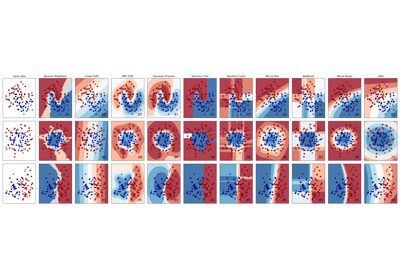Classifier comparison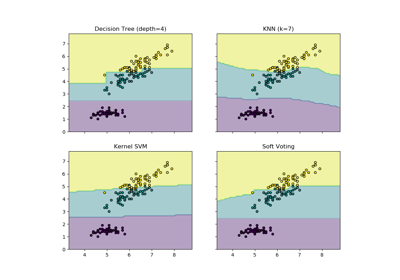Plot the decision boundaries of a VotingClassifier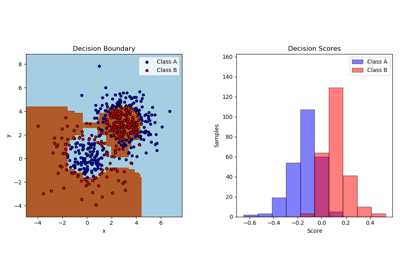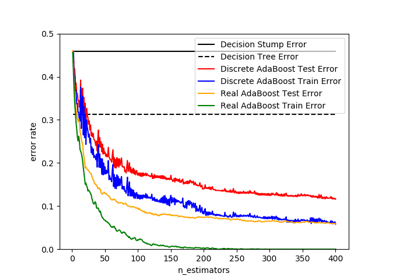Discrete versus Real AdaBoost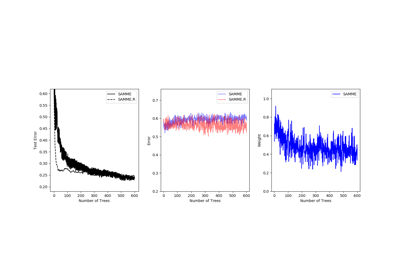Multi-class AdaBoosted Decision Trees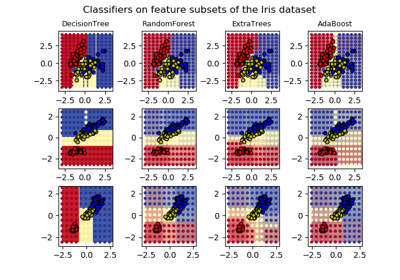Plot the decision surfaces of ensembles of trees on the iris dataset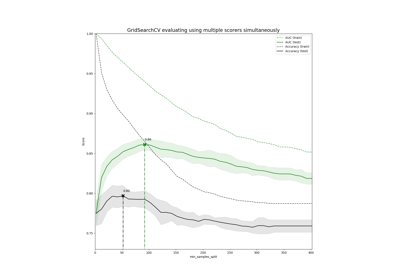Demonstration of multi-metric evaluation on cross_val_score and GridSearchCV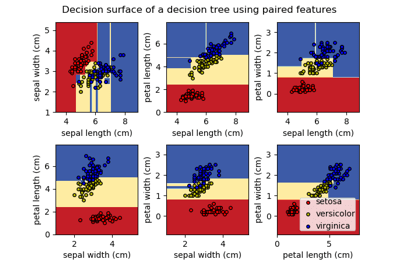Plot the decision surface of a decision tree on the iris datasetUnderstanding the decision tree structure

© 2007–2018 The scikit-learn developers